Click to Chat

1800-1023-196

+91-120-4616500

CART 0

• 0

MY CART (5)

Use Coupon: CART20 and get 20% off on all online Study Material

ITEM
DETAILS
MRP
DISCOUNT
FINAL PRICE
Total Price: Rs.

There are no items in this cart.
Continue Shopping• Kinematics & Rotational Motion
• OFFERED PRICE: Rs. 636
• View Details

Multiplication of Vectors

Table of Content

Multiplication of vector by a scalar

Multiplication of a vector by a vector

Dot product or scalar product

Some examples of dot product

Characteristics of dot product

Cross product or vector product

Characteristics of cross product

Related Resources

The vectors cannot be added algebraically, like scalar quantities. The angle between the two vectors plays an important role in addition of vectors. Similarly, angle between two vectors plays an important role when the two vectors are multiplied together.

Consider two quantities having magnitudes ‘3’ and ‘2’ respectively. If the quantities are scalars then then the result of their multiplication cannot be anything other than 6. We are at liberty to express these product as 3.2 = 6 or 32 = 6. If the two quantities are vector quantities, the result of their multiplication can have any value lying in between -6 and +6. The result depends upon the angle between them. Moreover we are not free to put a dot (.) or a cross () in between.

Multiplication of Vector by a Scalar

Let vector a is multiplied by a scalar m. If m is a positive quantity, only magnitude of the vector will change by a factor ‘m’ and its direction will remain same. If m is a negative quantity the direction of the vector will be reversed.

Multiplication of a Vector by a Vector

There are two ways in which two vectors can be multiplied together.(A) Dot Product or Scalar Product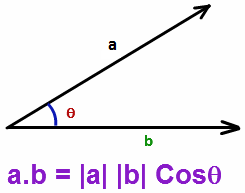The dot product of two vectors a and b is defined as the product of their magnitudes and the cosine of the smaller angle between the two.

It is written by putting a dot (.) between two vectors. The result of this product does not possess any direction. So, it is a scalar quantity. Hence it is also called a scalar product.
= || || cosθ = ab cosθ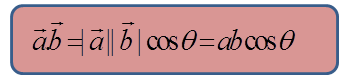where a and b are the magnitudes of the respective vectors and θ is the angle between them. The final product is a scalar quantity. If two vectors are mutually perpendicular then θ = 900 and cos 90 = 0, Hence, their dot product is zero.

Some Examples of Dot Product

Work (W):- Work is the dot product of the force () and displacement (). Work is scalar quantity.

W =  = Fs cosθ

Power (P):- Power is the dot product of force () nad velocity (). Power is also a scalar quantity.

P =

Characteristics of Dot Product

Dot product of two vectors obeys the following characteristics.

(i) Commutative:-

Dot product between two vectors is commutative in nature.

If  and  are two vectors,

then,

It means that the order of vectors in the product can be changed without affecting the result.

(ii) Distributive:-

Dot product of vector with the sum of a number of other vectors is equal to the sum of the dot products of the vector taken with other vectors separately.

Mathematically, it can be expressed as

(iii) Dot product in terms of rectangular component:-Here,(iv) Perpendicular vectors:-

For two perpendicular vectors  and , θ = 90º.

= || || cosθ = ab cos 90º = 0

Thus, the product of two non-zero vectors, which are perpendicular to each other, is always zero. This statement is known as condition of perpendicularity.

(v) Collinear vectors:-

(a) Parallel vectors:-

In this case, θ = 0º,

So,  = || || cosθ = ab cos 0º = ab

(b) Anti-parallel vectors:-

In this case,  θ = 180º,

= || || cosθ = ab cos 180º = -ab

Therefore, the dot product of collinear vectors is equal to the product of their magnitudes. It is positive if they are parallel and negative if they are anti-parallel. The statement is called condition of co-linearity.

(vi) Equal vectors:-

Vectors are equal if they possess same magnitude and direction, i.e.,

θ = 0º

So dot product of two equal vectors is given by

= |a||a| cos0º = A2

Dot product two equal vectors is equal to the square of the magnitude of the either.

Thus,

Problem 1:-

Find the angle between the vectors A and B where

= 2i + 3j + 3k,  = i + 2j - 3k

Solution :
We know that,

= |A||B| cosθ   where |A| = √(22 +32 + 32) = √22

|B| =√(12 + 22+ 32) = √14

Hence cosθ = ()/(|A||B|)

=((2i +3j +3k  )(i +2j -3k ))/(√22×√14)

=(2+6-9)/(2√77)=(-1)/(2√77)

Thus, θ = cos-1((-1)/(2√77))

Cross Product or Vector Product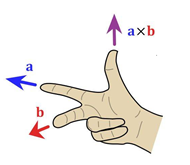Cross product of two vectors  and  is defined as a single vector  whose magnitude is equal to the product of their individual magnitudes and the sine of the smaller angle between them and is directed along tyhe normal to the plane containing  and .

x =  = ab sinθ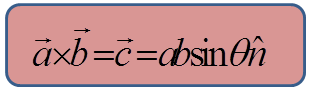Here  is the unit vector in a direction perpendicular to the plane containing  and .

Cross product of two vectors being a vector quantity is also known as vector product.

To specify the sense of the vector c, refer to the figure given below.Imagine rotating a right hand screw whose axis is perpendicular to the plane formed by vectors a and b so as to turn it from vectors a to b trough the angle θ between them. Then the direction of advancement of the screw gives the direction of the vector product vectors  and .

Examples:-

Angular momentum ():- Angular momentum  () which is the cross product of position vector () and momentum () is also a vector quantity.

= x

Characteristics of Cross Product

(i) Not commutative:-

Cross product of two vectors is not commutative in nature.

Thus,

(ii) Distributive:-

Cross product of  a vector with the sum of a number of vectors is equal to the sum of cross product of the vector taken with other vectors separately.

So,

(iii) Collinear vectors:-

(a) Parallel vectors:-

In this case, θ = 0º, sinθ = 0,

So, = a null vector

(b) Anti-parallel vectors:-

In this case,  θ =180º, sinθ = 0

Thus, again,  = a null vector

Therefore, cross product of two linear vectors is always a null vector (zero magnitude). The statement is known as condition of co-linearity.

(iv) Equal vectors:-

For equal vectors,

θ = 0º, sinθ = 0,

So, = a null vector

(v) Perpendicular vectors:-

For perpendicular vectors,

θ = 90º, sinθ = 1,

So, = ab

The magnitude of the cross product of two perpendicular vectors is equal to the product of their individual magnitudes.

(vi) Orthogonal unit vectors:-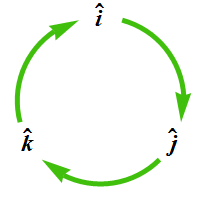In case of orthogonal unit vectors,

Problem 2:-

Obtain a unit vector perpendicular to the two vectors  = 2i + 3j + 3k,  = i – 2j + 3k

Solution:-

We know that  = AB sin θ n
n  = ()/ABsinθ
We have  = 17i-2j-7k
A = √29 B = √14
and θ = cos-1 8/(√14 √29) (Use concept of dot product to find θ).
From the above values we can find n
Solving we get,

n = (17i-2j-7k)/(√29 √14 sinθ)     where θ = cos-1 8/(√14 √29)The result of dot product of two vectors is a scalar quantity.

Dot product of the perpendicular vectors is always zero.

Dot product of two similar vectors is equal to the square of the magnitude of either of them.

Change in the order of vectors does not produce a change in the result of dot product.

Cross product of two vectors is a vector quantity.

Change in the order of vectors, in a cross product, results in the introduction of a negative sign.

Direction of cross product is always perpendicular to the plane containing two vectors.

While applying right thumb rule, for cross product, it must be ensured that both the vectors are directed away or directed towards the point of intersection.

Cross product of two linear vectors is always a null vector

Area of the parallelogram formed by the two vectors is equal to the magnitude of their cross-product.Question 1:-

If x = , then:

(a)  is perpendicular to  only.

(b)  is parallel to .

(c) is perpendicular to both  and .

(d) is parallel to both  and .

Question 2:-

Which of the following is correct?

(a)

(b)

(c)

(d)

Question 3:-

The angle between the two vectors  and  will be:

(a) zero    (b) 450    (c) 900    (d) 1800

Question 4:-

If || = || = || then angle between A and B will be:

(a) 900       (b) 1200        (c) 00    (d) 600

Question 5:-

A boat which has a speed of 5 km/h in still water crosses a river of width 1 km along the shortest possible path in 15 minutes. The velocity of the river was in km/h is

(a) 1                (b) 4               (c) √ 21           (d) 3Q.1
Q.2
Q.3
Q.4
Q.5

c

d

c

b

d

Related Resources

You might like to refer Scalar and Vectors.

For getting an idea of the type of questions asked, refer the  Previous Year Question Papers.

To read more, Buy study materials of Units & Dimensions comprising study notes, revision notes, video lectures, previous year solved questions etc. Also browse for more study materials on Physics here.### Course Features

• 731 Video Lectures
• Revision Notes
• Previous Year Papers
• Mind Map
• Study Planner
• NCERT Solutions
• Discussion Forum
• Test paper with Video Solution